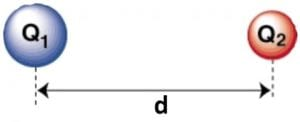Home » Coulomb’s Law

# Coulomb’s Law

« Back to Glossary Index

Coulomb’s Law is a formula that allows us to calculate the electric force generated between two electrical charges. Specifically, it allows calculating the strength and direction of electric force. This equation takes into account the strength of two charged pieces of matter (called “charges”) and the distance that separates them.[Image source: https://en.wikipedia.org/wiki/Charles-Augustin_de_Coulomb]The electric force is greater if the strength of the charges is greater. It is also greater if the charges are closer together. The electric force is weaker if the charges are weaker and if the distance between the charges is longer. Specifically, regarding distance, the electric force is inversely proportional to the square of the distance between the two charges. This, like the force of gravity, is called an “inverse square law.”

## Formula for Coulomb’s Law

Coulomb’s Law may be written:where F is the magnitude and direction of the electric force generated by the charges. The symbol Q1 is for the strength of one charge and Q2 is for the strength of the other.* The d is the distance between the charges. And k is Coulomb’s Law Constant. This is a specific number, a constant of nature dependent on the medium that the charges are immersed in. Coulomb’s Law Constant is a measure of the resistance of the medium to formation of an electrical field.Coulomb’s Law where Q1 and Q2 are two charged particle and d is the distance between them. [Image source: modification of http://cphysics.wikia.com/wiki/Coulomb%27s_Law]Coulomb’s Law works if the charges are limited to small points in space. Equations other than Coulomb’s Law must be used for extended bodies of charge, like when the charge lies along a line or covers a plane.

Coulomb’s Law was named after Charles-Augustin de Coulomb (1736-1806), the French engineer and physicist who developed the equation in around 1780.

*It is not known why Q has come down to us as the symbol for electric charge. One theory is that it stands for “quantity of charge.”

This site uses Akismet to reduce spam. Learn how your comment data is processed.